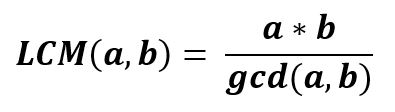# numpy.lcm() in Python

`numpy.lcm(arr1, arr2, out = None, where = True, casting = ‘same_kind’, order = ‘K’, dtype = None)` : This mathematical function helps user to calculate lcm value of |arr1| and |arr2| elements.Parameters :
arr1 / arr2 : [array_like]Input array.

Return : LCM of two or more numbers.

Code :

 `# Python program illustrating  ` `# lcm() method  ` `import` `numpy as np  ` ` `  `arr1 ``=` `[``120``, ``24``, ``42``, ``10``] ` `arr2 ``=` `[``2250``, ``12``, ``20``, ``50``] ` ` `  `print` `(``"arr1 : "``, arr1) ` `print` `(``"arr2 : "``, arr2) ` ` `  `print` `(``"\nlcm of arr1 and arr2 : "``, np.lcm(arr1, arr2)) ` `print` `(``"\nlcm of arr1 and 10   : "``, np.lcm(arr1, ``10``)) ` `                    `

Output :

```arr1 : [120, 24, 42, 10]
arr2 : [2250, 12, 20, 50]

lcm of arr1 and arr2 : [9000,   24,  420,   50]

lcm of arr1 and 10   : [120, 120, 210,  10]
```

My Personal Notes arrow_drop_upAspire to Inspire before I expire

If you like GeeksforGeeks and would like to contribute, you can also write an article using contribute.geeksforgeeks.org or mail your article to contribute@geeksforgeeks.org. See your article appearing on the GeeksforGeeks main page and help other Geeks.

Please Improve this article if you find anything incorrect by clicking on the "Improve Article" button below.

Article Tags :

Be the First to upvote.

Please write to us at contribute@geeksforgeeks.org to report any issue with the above content.